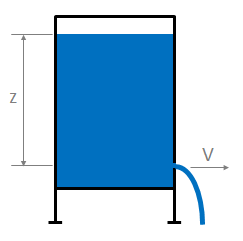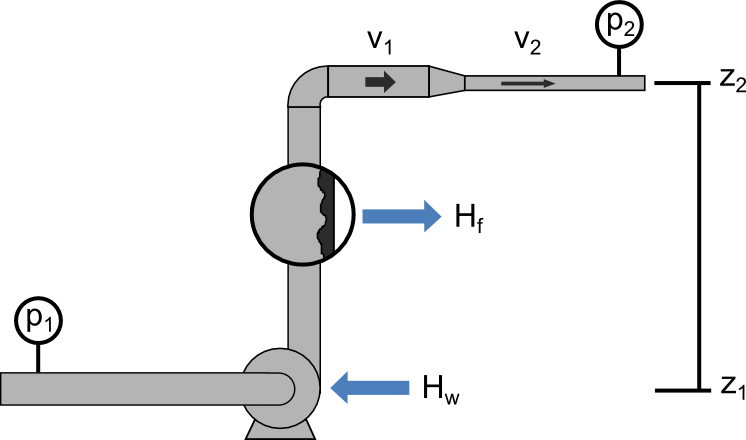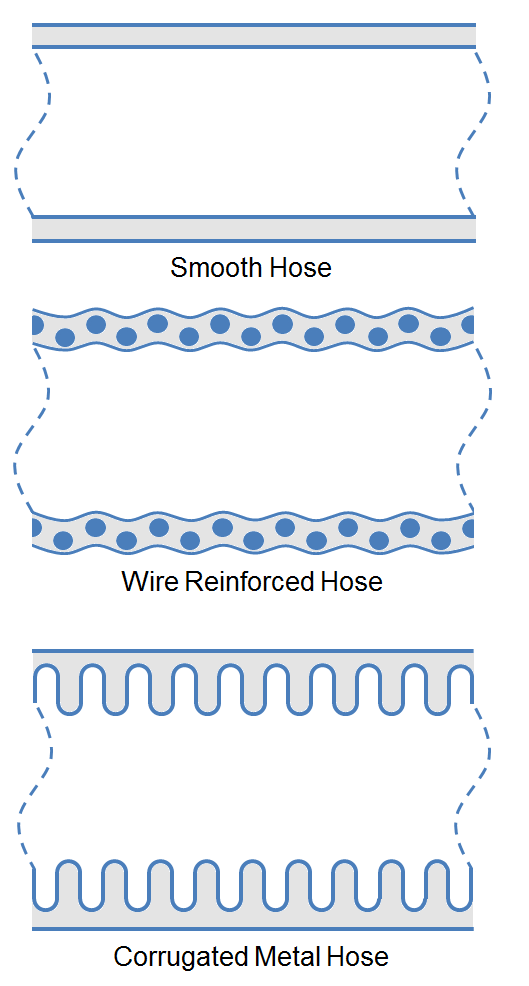f
Neutrium

# Welcome to Neutrium

Neutrium is a knowledge base of engineering topics, centred mainly around chemical engineering design challenges faced by engineers in their daily work. We created Neutrium to bridge the gap between theory and practice. Feel free to ask a question, leave feedback or take a look at one of our in-depth articles.

Bulk Modulus of Elasticity of Liquids

The bulk modulus or elasticity is a measure of the resistance to compression of a substance. This article presents the bulk modulus for various liquids.

Comparing Volumetric Flow Rates for Equivalent Pressure Loss

Standard volumetric flow rates of a fluid are often used to describe the capacity of a vent or pressure relief device. To determine how this capacity compares for another fluid under different pressure and temperature conditions a conversion must be made on the basis of equivalent pressure loss. This article describes the method for calculating the volumetric flow rate of a gas which will give the equivalent pressure drop to another gas through a fixed restriction such as a vent.

Torricelli's LawThis article presents Torricelli’s law, a simplified method of estimating the velocity of fluid passing through an open orifice under static pressure.

Bernoulli's EquationBernoulli’s Principle is an important observation in fluid dynamics which states that for an inviscid flow, an increase in the velocity of the fluid results in a simultaneous decrease in pressure or a decrease in the fluid’s potential energy. This principle is often represented mathematically in the many forms of Bernoulli’s equation. This article presents some useful forms of Bernoulli’s Equations and their simplifying assumptions.

Exponent LawsAlgebraic expressions can often be simplified and subsequently solved through the use of the exponent laws (also called laws of indices or power laws). These laws allow an equation to be manipulated into a form which provides enhanced readability or opens up potential simplifications and substitutions. This article provides a reference for these laws.

Algebraic FactoringWhen developing algebraic expressions it is often useful to factorise the expression to familiar components. Through factorisation, one can increases both the readability and manipulability of the expression. This article summarises the common algebra factorisation relationships.

Laws of Thermodynamics

The laws of thermodynamics are fundamental laws which describe the behaviour of heat and work in a thermodynamic system. These laws forbid phenomena such as perpetual motion machines, a hypothetical machine the development of which was pursued during the industrial revolution.

Specific Energy and Energy Density of Fuels

Specific energy and energy density are useful measures of the energy that will be released from a given weight or volume of fuel when it is burned. This article provides a quick reference for common values of specific energy and energy density.

Pressure Loss in HosesThe Pressure loss through a hose is often approximated using coarse heuristics, but utilization of more accurate correlations increase the efficiency of pump and piping designs. This article presents more accurate methods to estimate the pressure loss in various type of hoses using multiples of the pipe length. Methods of estimating pressure loss caused by couplings, curves and coiled hose are also detailed.

Heat of Combustion

The heat of combustion is the energy liberated when a substance undergoes complete combustion, at constant pressure usually in an environment with excess Oxygen. The heat of combustion is utilised to quantify the performance of a fuel in a combustion system such as furnaces, power generation turbines and motors. This article describes the heat of combustion and provides a list of heats of combustion for commons fuels and fuel components.